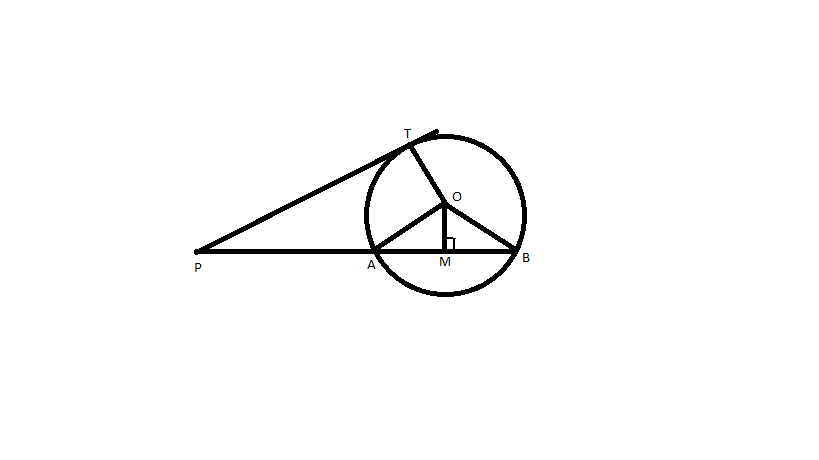Courses
Courses for Kids
Free study material
Free LIVE classes
MoreLIVE
Join Vedantu’s FREE Mastercalss

# If PAB is a secant to a circle of Centre O intersecting the circle of A and B and PT is tangent segment, then prove that-${\text{PA}} \times {\text{PB = P}}{{\text{T}}^2}$Verified
360.9k+ views
Hint – In this question first make a circle then draw a tangent and a secant from an external point of the circle and also, draw a perpendicular line from the Centre of the circle to the secant, then apply Pythagoras theorem .

Complete step-by-step solution -

Given Data, PAB is a secant, Centre of circle O, PT is a tangent
We make a construction that is OM ⊥ AB, OA, OP, OT, Ob are joined.Here PAB is secant intersecting the circle with Centre O at A and B and a tangent PT at T.
Now PA = PM – AM
PB = PM + MB
As we know AM = BM (perpendicular drawn from the Centre of the circle to a chord is also a bisector of chord)
PA.PB = (PM – AM) × (PM + AM) = ${\text{P}}{{\text{M}}^2} - {\text{A}}{{\text{M}}^2}{\text{ }} \to {\text{ Equation 1}}$
Also from the diagram OM ⊥ AB
We can apply Pythagoras theorem in ∆OMP
${\text{P}}{{\text{M}}^2} = {\text{O}}{{\text{P}}^2} - {\text{O}}{{\text{M}}^2}$
We can apply Pythagoras theorem in ∆OMA
${\text{A}}{{\text{M}}^2} = {\text{O}}{{\text{A}}^2} - {\text{O}}{{\text{M}}^2}$
Put these values in Equation 1, we get
PA.PB = ${\text{P}}{{\text{M}}^2} - {\text{A}}{{\text{M}}^2}$
PA.PB = (${\text{O}}{{\text{P}}^2} - {\text{O}}{{\text{M}}^2}$) – (${\text{O}}{{\text{A}}^2} - {\text{O}}{{\text{M}}^2}$)
PA.PB = ${\text{O}}{{\text{P}}^2} - {\text{O}}{{\text{A}}^2}$
PA.PB = ${\text{O}}{{\text{P}}^2} - {\text{O}}{{\text{T}}^2}{\text{ }} \to {\text{ Equation 2}}$
We get ${\text{P}}{{\text{T}}^2}{\text{ = O}}{{\text{P}}^2} - {\text{O}}{{\text{T}}^2}$
PA.PB = ${\text{P}}{{\text{T}}^2}$